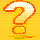## General Question# Brain Teaser - How does this work?

Asked by LostInParadise (29640) March 27th, 2009

This may be a bad time of year for this, but I am hoping there is enough tease in this to catch someone’s attention.

Given a list of numbers, for example, 3, 4, 6, 8 and 9, remove two of the numbers a and b from the list and replace them with a*b + a + b. Keep doing this until one number remains. No matter what order you do this in the answer will be the same, in this case 12,599.

Hint: a*b + a + b can almost be factored.

I will provide the short answer and also use this problem as an introduction to the concept of isomorphism, which tells when two operations are structually identical.

Observing members: 0Composing members: 0Starting with n numbers a(1), a(2), ..., a(n) we will always end up with (a(1)+1) ( a(2)+1)...(a(n)+1) -1.

The reason is that ab+a+b = (a+1)(b+1) – 1

One way to prove the above statement is by induction on n: Lets say in the step right before the last step we are left with two numbers a and b. We know a and b are results of this operation on k and n-k numbers for some k < n. So by induction hypothesis a = (a(1)+1)...(a(k)+1) -1 and b =(a(k+1)+1)...(a(n)+1) -1

Calculating (a+1)(b+1)-1 proves the statement for n, which shows the statement is true by induction on n.

BonusQuestion (1483)“Great Answer” (1) Flag as…Another way of proving that statement is to show in each step the product of (a(i)+1) does not change. Meaning that if we do the operation on a(1),...,a(n) and we get b(1),...,b(n-1) then

(a(1)+1) .... (a(n)+1) = (b(1)+1) .... (b(n-1)+1)

Again the reason is that we replace a , b by (a+1)(b+1)-1.

Therefore if we finally get to a single number x , then we should have: x+1 is the same as the product of (a(i)+1) which means x = ( product of (a(i)+1) ) -1

BonusQuestion (1483)“Great Answer” (0) Flag as…@BonusQuestion , you got it right. I would like to show another way of looking at it that is not quite so easy to explain as I had hoped, but I can give a rough idea of how it works.

Suppose we define an operation & as
a & b = a * b + a + b. Then what we want to show is that the order in which & is applied does not matter and to do that it is sufficient to show & is commutative and associative.

We have a & b + 1 = (a+1)*(b+1). If we let T(x) = x + 1 then we get T(a & b) = T(a)*T(b). This is an isomorphic relationship, meaning that the internal structure of & is the same as *. You can think of T as a kind of reflection. On one side you get a & b = c and on the other you get T(a) * T(b) = T©. What happens on one side matches up to what happens on the other if you replace x with T(x) and & with *. For example T(a & b) = T(a) * T(b) and T(b & a) = T(b) * T(a), so & is commutative because * is. Similarly for associativity.

LostInParadise (29640)“Great Answer” (1) Flag as…or